## 17.8 Examples using $$z$$-scores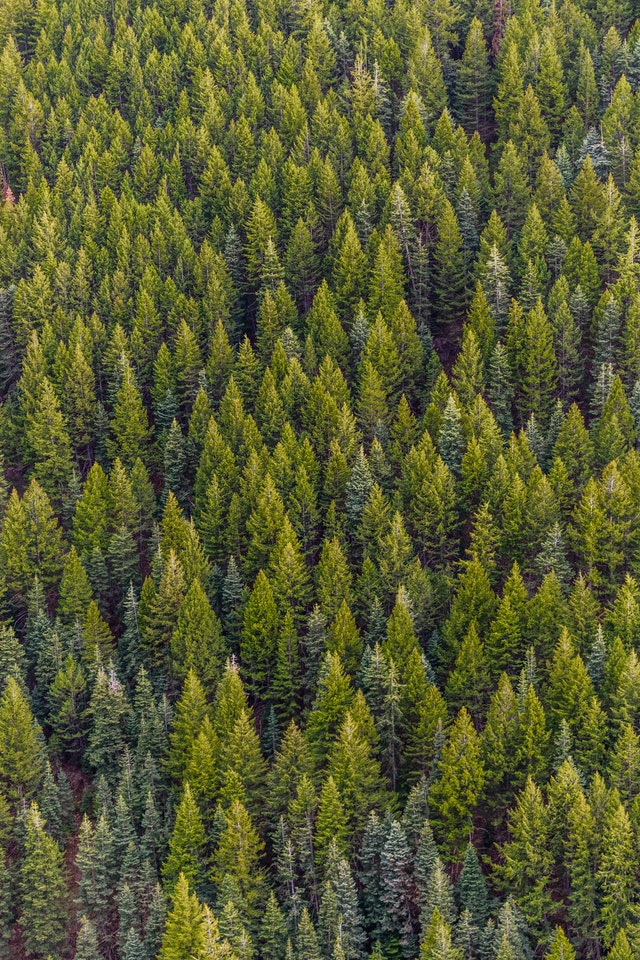Example 17.7 (Normal distributions) simulated mechanized forest harvesting systems .

As part of their study, they assumed that the specific trees in their study would vary in diameter, with

• a normal distribution; with
• a mean of $$\mu=8.8$$ inches; and
• a standard deviation of $$\sigma=2.7$$ inches.
Using this model, what is the probability that a tree has a diameter greater than than 6 inches?

• Draw a normal curve, and mark on 6 inches (Fig. 17.7, top panel).
• Shade the region corresponding to ‘greater than 6 inches’ (Fig. 17.7, bottom panel).
• Compute the $$z$$-score using Eq. (17.1). Here, $$x=6$$, $$\mu=8.8$$, $$\sigma=2.7$$, so $$\displaystyle z = (6 - 8.8)/2.7 = -2.8/2.7 = -1.04$$ to two decimal places.
• Use tables: The probability of a tree diameter shorter than 6 inches is $$0.1492$$. (The tables always give area less than the value of $$z$$ that is looked up.)
• Compute the answer: Since the total area under the normal distribution is one, the probability of a tree diameter greater than 6 inches is $$1 - 0.1492 = 0.8508$$, or about 85%.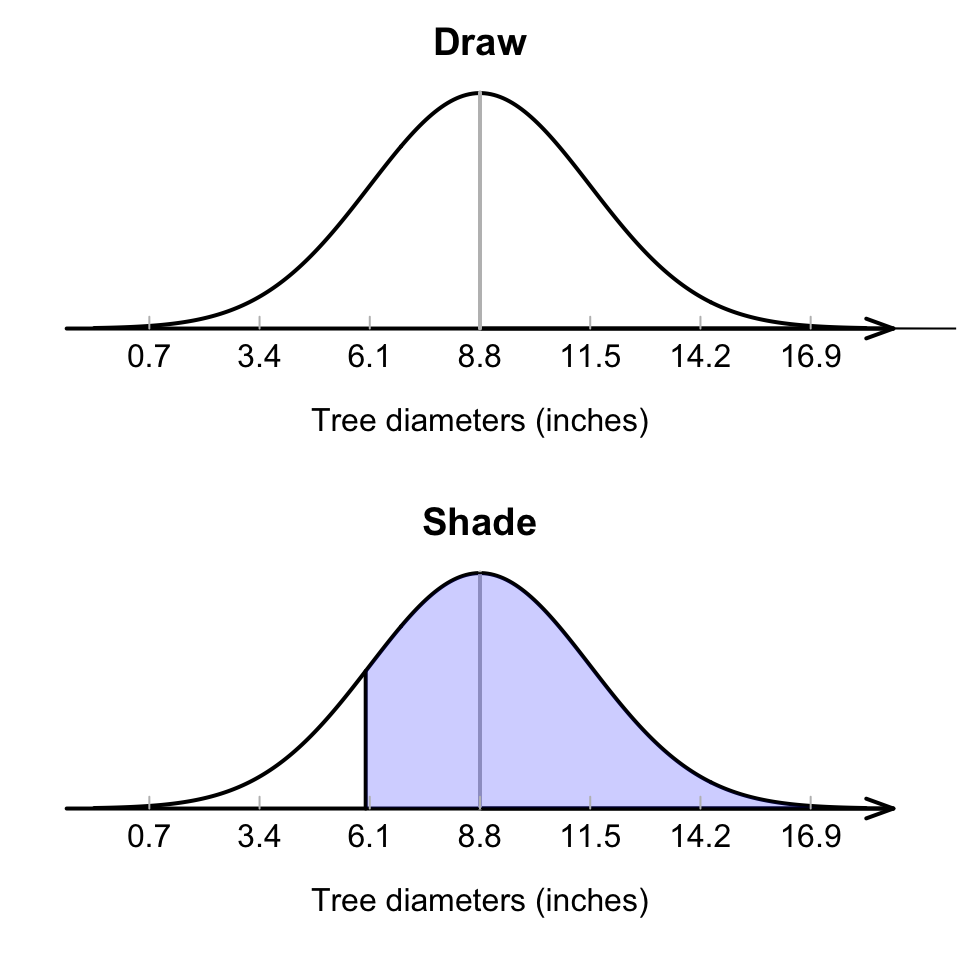FIGURE 17.7: What proportion of tree diameters are greater than 6 inches?

The normal-distribution tables in the Appendix always provide area to the left of the $$z$$-scores that is looked up. Drawing a picture of the situation is important: it helps visualise how to get the answer from what the table give us.

Remember: The total area under the normal distribution is one.

Think 17.1 (Drawing diagrams) Match the diagram in Fig. 17.8 with the meaning for the tree-diameter model (recall: $$\mu=8.8$$ inches):

1. Tree diameters greater than 11 inches.
2. Tree diameters between 6 and 11 inches.
3. Tree diameters less than 11 inches.
4. Tree diameters between 3 and 6 inches.
1: B; 2: C; 3: D; 4: A.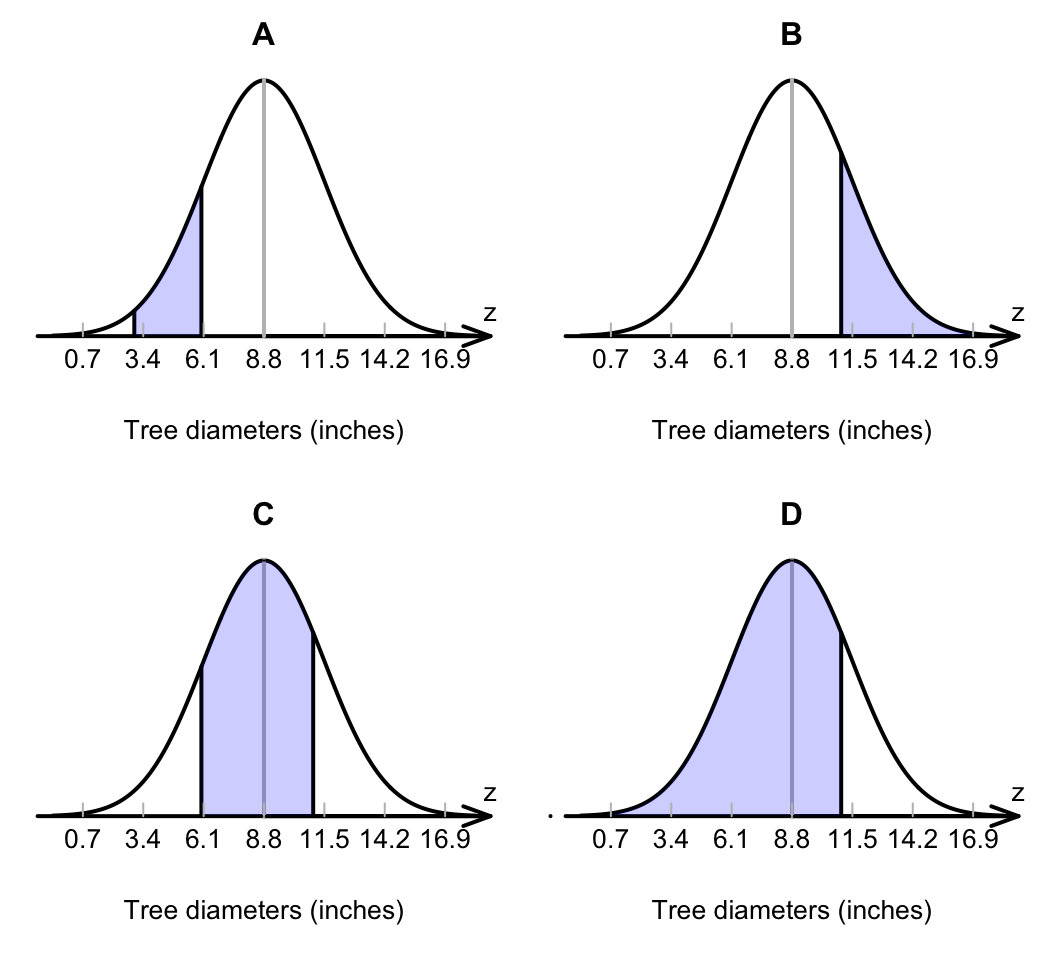FIGURE 17.8: Match the diagram with the description

Example 17.8 (Normal distributions) Using the model for tree diameters in Example 17.7 , what is the probability that a tree has a diameter between 6 and 11 inches?

First, draw the situation, and shade ‘between 6 and 10 inches’ (Fig. 17.9). Then, compute the $$z$$-scores for both tree diameters:

\begin{align*} \text{6 inches: } &z = \displaystyle \frac{6 - 8.8}{2.7} = -1.04;\\[6pt] \text{11 inches: } &z = \displaystyle \frac{11 - 8.8}{2.7} = 0.81. \end{align*} Table B can then be used to find the area to the left of $$z = -1.04$$, and also the area to the left of $$z = 0.81$$. However, neither of these provide the area between $$z = -1.04$$ and $$z = 0.81$$ (Fig. 17.10).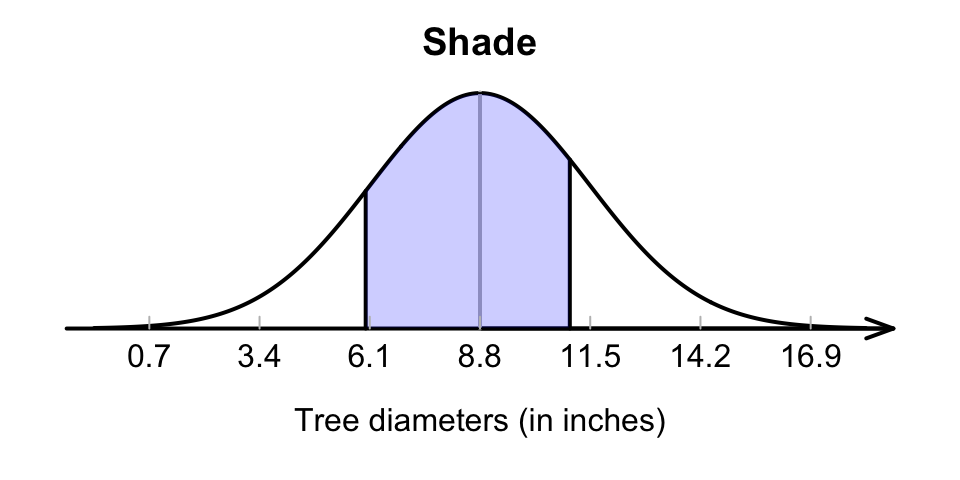FIGURE 17.9: What proportion of tree diameters are between 6 and 11 inches?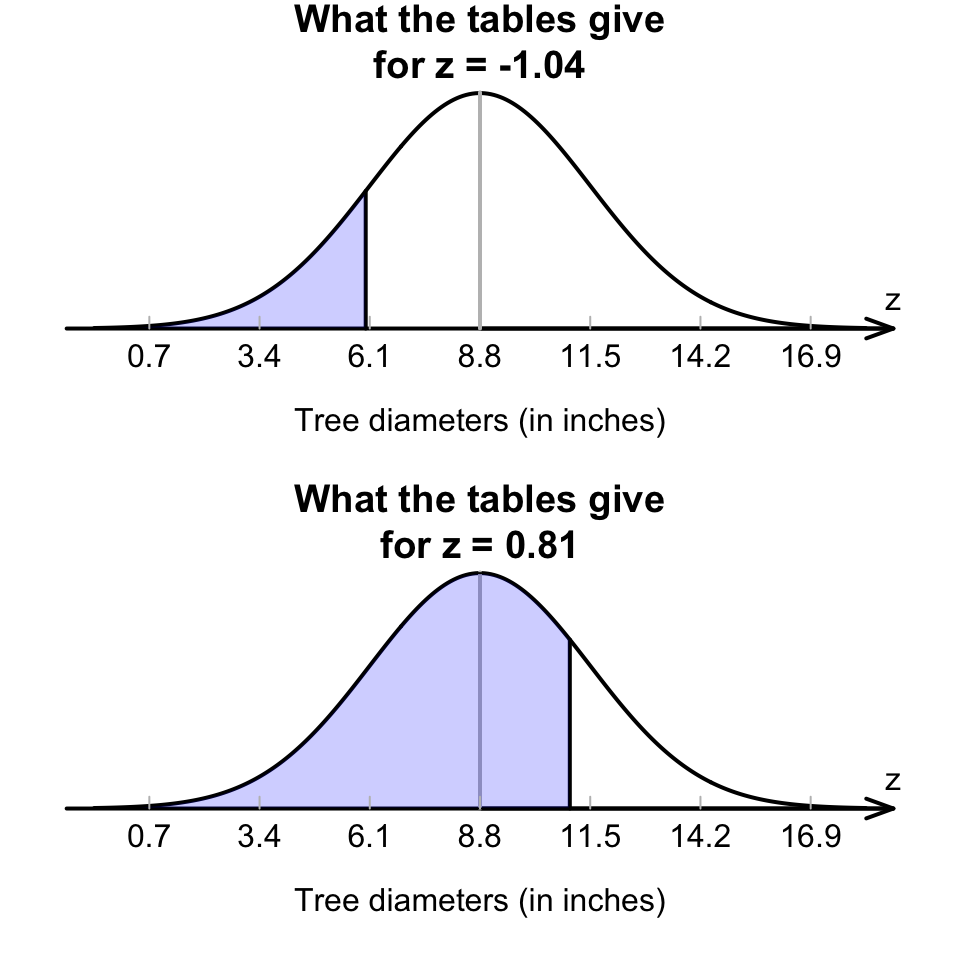FIGURE 17.10: What proportion of tree diameters are between 6 and 11 inches? The two shaded areas given are what we find by using the tables with $$z=-1.04$$ and $$z=0.81$$, but neither give us the area we are seeking

Looking carefully at the areas from the tables and the area sought, that area between the two $$z$$-scores is

$0.7910 - 0.1492 = 0.6418;$ see the animation below. The probability that a tree has a diameter between 6 and 11 inches is about 0.6418, or about 64%.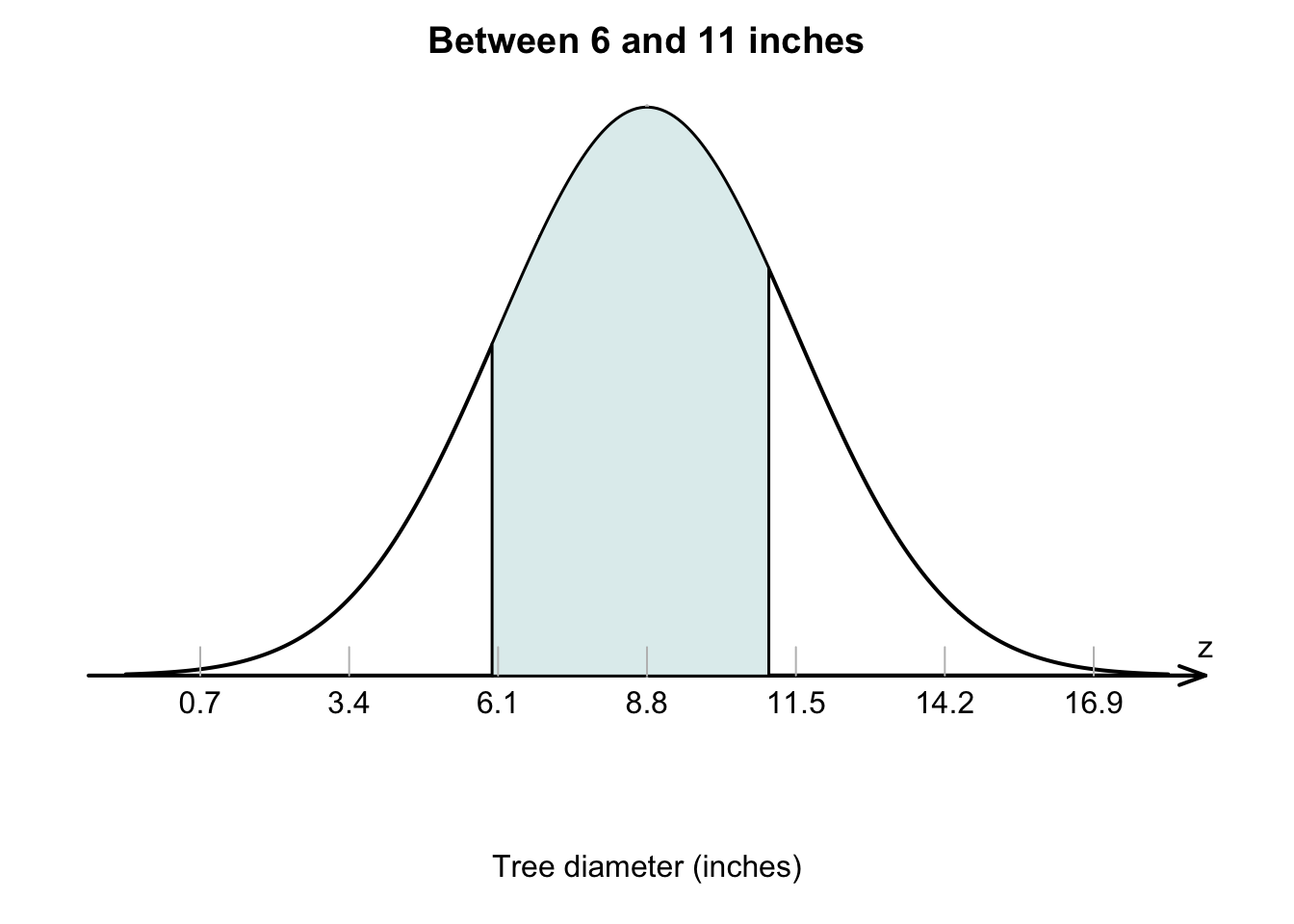Click on the hotspots in the following image, to see what the areas under the normal curve mean.

### References

Aedo-Ortiz DM, Olsen ED, Kellogg LD. Simulating a harvester-forwarder softwood thinning: A software evaluation. Forest Products Journal. 1997;47(5):36–41.
Devore JL, Berk KN. Modern mathematical statistics with applications. Thomson Higher Education; 2007.